# Change Nickname

Current Nickname:

Certifications

Certificate Issued at Valid until Serial No. File
Serial No. Valid until

Not having one? Apply now! >>>

Invoice

ProductName CreateTime ID Price File
ProductName CreateTime ID Price File

# Minimum Spanning Tree

## Overview

Minimum Spanning Tree algorithm can calculate the minimum connected subgraphs of each connected component of a graph, and return the edges in these minimum connected subgraphs in the form of path. Minimum spanning tree is one of the fundamental concepts in graph theory that has important application in path optimization and lowest cost solutions.

Different minimum spanning trees may exist for one graph, Ultipa's MST algorithm only calculates and returns one of them.

## Basic Concept

### MST

For a connected graph with `n` nodes, its minimum connected subgraph consists of `n` nodes and `n-1` edges of the graph; these edges connect all `n` nodes, and if removes any one edge, the `n` nodes will not be connected anymore; these `n-1` edges form a MST of the graph.

The connected graph below has 11 nodes, the 10 edges highlighted in green form a MST of this graph: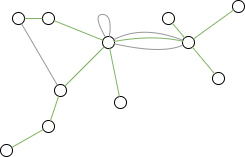Minimum connected subgraph must not have 1) circle, 2) self-loop edge and 3) multiple edges between two nodes. Redraw this graph according to these features as below: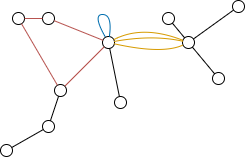One MST can be obtained by 1) removing one edge in the red circle, 2) removing the blue self-loop edge and 3) keeping any one edge among the 3 yellow edges. There are `4 * 3 = 12` possible MSTs of this graph.

### Weighted MST

Weighted MST is calculated by adding all edge weights of the MST up and keep the MST with the lowest weight sum. The number of MSTs may reduce when taking weight into account, but there still may exist multiple MSTs.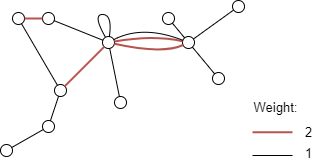As the above graph shows, given that the weight of red edge is 2 and the weight of black edge is 1, then this graph has 2 weighted MST solutions, each are indicated in green, and the sums of weight are both `9 *1 + 1 * 2 = 11`.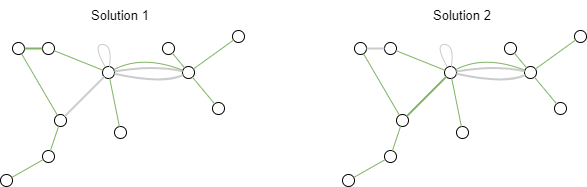Ultipa's MST algorithm only calculate weighted MST for the real applications. If ignores edge weight, then any spanning tree is a minimum spanning tree.

### Start Node of MST

For connected graph, the number of edges in MST = the number of nodes in graph - 1; that is to say, for any graph, the number of nodes in graph = the number of edges in MST + the number of connected components.

Only when a start node is specified for a connected component, the MST algorithm can calculate the MST for that connected component. Connected components without a specific start node will be ignored. It is sufficient to specify one start node for each connected component, more than one is invalid. If the specified node is a lonely node, it will not take any effect too.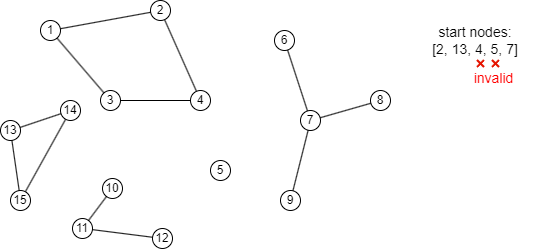As the above graph shows, when specifying the start node sequence `[2, 13, 4, 5, 7]`: node 4 is invalid because it is in the same connected component with node 2; node 5 is invalid because it is a lonely node. The algorithm calculates MST for each connected component in the order of `[2, 13, 7]`; the connected component which contains node 10, 11 and 12 will not participate in the calculation because it has no start node specified.

## Special Case

### Lonely Node, Disconnected Graph

Lonely node does not participate in the calculation of MST.

In disconnected graph, MST is calculated for each connected component which has a start node specified.

### Self-loop Edge

Self-loop edge does not participate in the calculation of MST.

### Directed Edge

For directed edges, MST algorithm ignores the direction of edges but calculates them as undirected edges.

## Results and Statistics

Take the graph below as an example, node 1 is an electric center, node 2~8 are 7 villages around the center, the number of kilometers marked on each edge represents the distance between two locations (i.e. the required cable length), run the MST algorithm to find the lowest cost cabling solution: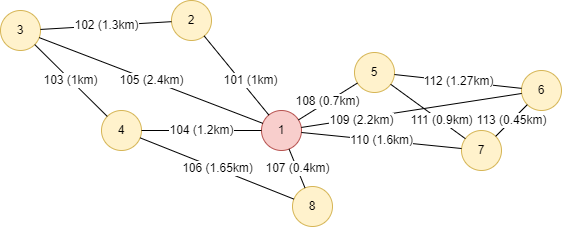Algorithm results: Specify node 1 as the start node, and use the property distance as weight, return the MST (1-hop path)

8 ---- 1
1 ---- 5
5 ---- 7
7 ---- 6
1 ---- 2
4 ---- 1
3 ---- 4

So the lowest cost cabling solution is as below: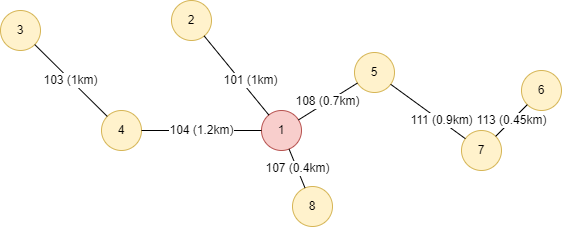Algorithm statistics: N/A

## Command and Configuration

• Command: `algo(mst)`
• Configurations for the parameter `params()`:
Name Type
Default
Specification
Description
ids / uuids []_id / []_uuid / / IDs or UUIDs of the start nodes of MST; all nodes to be specified if not set
edge_schema_property []`@<schema>?.<property>` / Numeric edge property, LTE needed; mandatory Edge weight property/properties, schema can be either carried or not; edge with multiple specified properties is calculated by the property with the lowest weight; edge without any specified property does not participate in the calculation
limit int -1 >=-1 Number of results to return; return all results if sets to -1 or not set

Example: Start from node UUID = 1, use edge property distance as weight to calculate MST, i.e. use the 'shortest distance' to connect all nodes

``````algo(mst).params({
uuids: ,
edge_schema_property: distance
}).stream() as a return a
``````

## Algorithm Execution

#### 1. File Writeback

Configuration
Data in Each Row
Description
filename `_id--[_uuid]--_id` startNode ID -- edge UUID -- endNode ID

Example: Start from node UUID = 1, use edge property distance as weight to calculate MST, write the algorithm results back to file named solution

``````algo(mst).params({
uuids: ,
edge_schema_property: distance
}).write({
file:{
filename: "solution"
}
})
``````

#### 2. Property Writeback

Not supported by this algorithm.

#### 3. Statistics Writeback

This algorithm has no statistics.

### Direct Return

Alias Ordinal
Type
Description
Column Name
0 []`path` 1-hop path that forms the MST, namely the startNode, edge and endNode `_uuid` --`_uuid`-- `_uuid` in one column

Example: Start from node UUID = 1, use edge property distance as weight to calculate MST, define algorithm results as alias named mst, and return the results

``````algo(mst).params({
uuids: ,
edge_schema_property: distance
}) as mst
return mst
``````

### Streaming Return

Alias Ordinal
Type
Description
Column Name
0 []`path` 1-hop path that forms the MST, namely the startNode, edge and endNode `_uuid` --`_uuid`-- `_uuid` in one column

Example: Start from node UUID = 1, use edge property distance as weight to calculate MST, return the sum of distance of all edges in the MST

``````algo(mst).params({
uuids: ,
edge_schema_property: distance
}).stream() as mst
with pedges(mst) as mstUUID
find().edges(mstUUID) as edges
return sum(edges.distance)
``````

### Real-time Statistics

This algorithm has no statistics.